CRR Tool
Capital Requirements Regulation (CRR)
Article 325ay

# Article 325ay - Vega and curvature risk correlations

Status
In force
Selected consolidated version from
30/09/2021
Search all articles and keywords within this legal act

Article 325ay

Vega and curvature risk correlations

1.

Between vega risk sensitivities within the same bucket of the general interest rate risk (GIRR) class, the correlation parameter ρkl shall be set as follows:.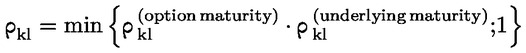where: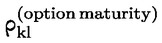shall be equal to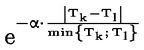where α shall be set at 1 %, Tk and Tl shall be equal to the maturities of the options for which the vega sensitivities are derived, expressed as a number of years; and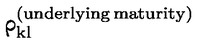is equal to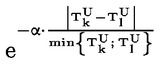, where α is set at 1 %,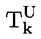and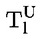shall be equal to the maturities of the underlyings of the options for which the vega sensitivities are derived, minus the maturities of the corresponding options, expressed in both cases as a number of years.
2.

Between vega risk sensitivities within a bucket of the other risk classes, the correlation parameter ρkl shall be set as follows: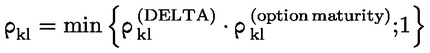where: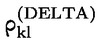shall be equal to the delta intra-bucket correlation corresponding to the bucket to which vega risk factors k and l would be allocated; andshall be set in accordance with paragraph 1.
3.
With regard to vega risk sensitivities between buckets within a risk class (GIRR and non-GIRR), the same correlation parameters for γbc, as specified for delta correlations for each risk class in Section 4, shall be used in the vega risk context.
4.
There shall be no diversification or hedging benefit recognised in the standardised approach between vega risk factors and delta risk factors. Vega risk charges and delta risk charges shall be aggregated by simple summation.
5.
The curvature risk correlations shall be the square of corresponding delta risk correlations ρkl and γbc referred to in Subsection 1.